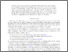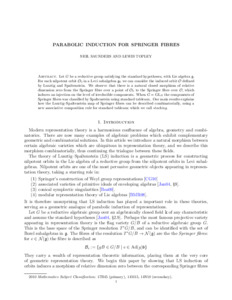# Parabolic induction for springer fibres

Saunders, Neil and Topley, Lewis (2023) Parabolic induction for springer fibres. Proceedings of the American Mathematical Society. ISSN 0002-9939 (Print), 1088-6826 (Online) (doi:https://doi.org/10.1090/proc/16361)Preview
PDF (AAM)
38432_SAUNDERS_Parabolic_induction_for_springer_fibres.pdf - Accepted Version

## Abstract

Let $G$ be a reductive group satisfying the standard hypotheses, with Lie algebra g. For each nilpotent orbit $\mathcal_{O}_0$ in a Levi subalgebra \mathfrak{g}_{0} we can consider the induced orbit $\mathcal{O}$ defined by Lusztig and Spaltenstein. We observe that there is a natural closed morphism of relative dimension zero from the Springer fibre over a point of $\mathcal{O}_{0}$ to the Springer fibre over O, which induces an injection on the level of irreducible components. When $G = GL_N$ the components of Springer fibres was classified by Spaltenstein using standard tableaux. Our main results explains how the Lusztig–Spaltenstein map of Springer fibres can be described combinatorially, using a new associative composition rule for standard tableaux which we call stacking.

Item Type: Article springer fibres; representation theory; algebraic geometry Q Science > QA Mathematics > QA75 Electronic computers. Computer scienceT Technology > T Technology (General) Faculty of Engineering & ScienceFaculty of Engineering & Science > School of Computing & Mathematical Sciences (CMS) 05 May 2023 10:49 http://gala.gre.ac.uk/id/eprint/38432View Item